Courses

# Additional Questions Solutions- Circles Class 9 Notes | EduRev

## Class 9 Mathematics by Full Circle

Created by: Full Circle

## Class 9 : Additional Questions Solutions- Circles Class 9 Notes | EduRev

The document Additional Questions Solutions- Circles Class 9 Notes | EduRev is a part of the Class 9 Course Class 9 Mathematics by Full Circle.
All you need of Class 9 at this link: Class 9

Question 1. In the figure, OD is perpendicular to chord AB of a circle whose centre is O. If BC is a diameter, prove that CA = 2OD.
Solution:
We have a circle whose centre is O. BC is a diameter and AB a chord such that OD ⊥ AB. Let us join AC.
∵ The perpendicular from centre of a circle to a chord bisects the chord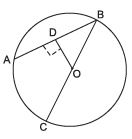∴ D is the mid-point of AB
∵ O is the mid-point of the diameter BC
∴ OD is the line segment joining the mid-points of two sides of ΔABC.
∴ OD is half of the third side of ΔABC.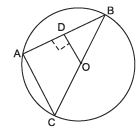i.e. OD =(1/2)AC
or 2OD = AC

Question 2. l is a line intersecting two concentric circles having common centre O, at A, B, C and D. Prove that AB = CD.
Solution:
We have two concentric circles with common centre O.
Line l intersects these circles at A, B, C and D.
Let us draw OP ⊥ ℓ For the inner circle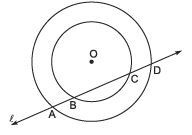∵ OP ⊥ BC                 [construction]
∴ P is the mid-point of BC                 [∵ Perpendicular from the centre to a chord bisects the chord]
∴ PB = PC                 …(1)
For the outer circle.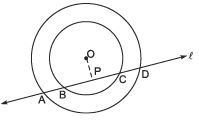∴ PA = PD                ..(2)
Subtracting (1) from (2), we have PA - PB = PD - PC
⇒ AB = CD

Question 3. AB and CD are equal chords of a circle whose centre is O. When produced, these chords meet at E. Prove that EB = ED.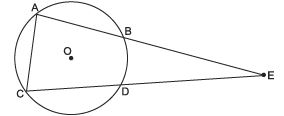Solution: We have a circle with centre O. Chord AB = chord CD and on production, AB and CD meet at E.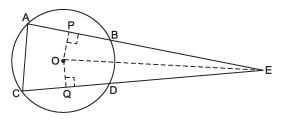Let us join OE and draw OP ⊥ AB and OQ ⊥ CD.
∵ In a circle, equal chords are equidistant from the centre.
∴ OP = OQ.                [∵ AB = CD]
Now, in right ΔOPE and right ΔOQE, we have OP = OQ                 [Proved],
OE = OE                [Common]
∵ Using RHS criterion, ΔOPE ≌ ΔOQE
⇒ P E = QE                 [c.p.c.t.]           …(1)
But AB = CD                [Given]
⇒ (1/2) AB = (1/2)CD or PB = QD                 [∵ OP ⊥ AB and OQ ⊥ CD] …(2)
Subtracting (2) from (1), we have PE ∠ PB = QE ∠ QD
⇒ EB = ED

Question 4. If O be the centre of the circle, find the value of ‘x’ in each of the following figures.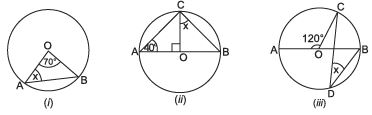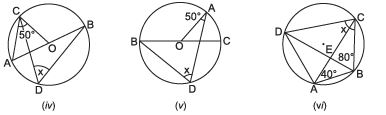Solution: (i) ∵ OA = OB                 [Radii of the same circle]
∴ ∠A = ∠B                 [Angles opposite to equal side in a triangle are equal]
In ΔABC, ∠A + ∠B + ∠O = 180º
∴ x + x + 70º = 180º                 [∵ ∠O = 70º (given) and ∠A = ∠B]
⇒ 2x + 70º = 180º
⇒ 2x = 180º - 70º = 110º
⇒ x= (1100/2)= 55º
Thus, x = 55°

(ii) In ΔAOC, ∠A + ∠ACO + ∠AOC = 180º
⇒ 40º + ∠ACO + 90º = 180º
⇒ ∠ACO = 180º - 40º - 90º = 50º
∵ AB is a diameter.
∴ ∠ACB = 90º                 [Angle in a semicircle]
∴ 50º + x = 90º
⇒ xº = 90º - 50º = 40º
Thus, x = 40º

(iii) ∵ ∠AOC + ∠COB = 180º                 [Linear pairs]
∴ 120º + ∠COB = 180º
⇒ ∠COB = 180º - 120º = 60º
∵ The arc CB is subtending ∠COB at the centre and ∠CDB at the remaining part.
∴ ∠CDB = (1/2)∠COB
⇒ x= (1/2)(60º) = 30º
⇒ x = 30º

(iv) In ΔAOC,
∵ AO = OC                 [Radii of the same circle]
∴ ∠OAC = ∠OCA                [Angles opposite to equal sides are equal]
⇒ ∠OAC = 50º
∴ Exterior ∠COB = 50º + 50º = 100º.
Now, the arc BC is subtending ∠BOC at the centre and ∠BDC at the remaining part of the circle.
∴ ∠BDC = (1/2)∠BOC
⇒ x= (1/2)(100º) = 50º
Thus, x = 50º

(v) In ΔOAC, OA = OC                 [Radii of the same circle]
∴ ∠AOC = ∠ACO                [∵ Angles opposite to equal sides are equal]
Now, ∠AOC + ∠ACO + ∠OAC = 180º
⇒ ∠AOC + ∠ACO + 50º = 180º
⇒ ∠AOC + ∠ACO = 180º - 50º = 130º
⇒ ∠AOC = ∠ACO = (1300/2) = 65º
Now, ∠AOB + ∠AOC = 180º                [Linear pairs]
∴ ∠AOB + 65º = 180º
⇒ ∠AOB = 180º - 65º = 125º
∵ The arc AB subtends ∠AOB at the centre and ∠ADB at the remaining part of the circle.
⇒ x= (1/2)(125º) = 62 (1/2)
∴ x= 62(1/2)

(vi) ∵ ∠BDC = ∠BAC                [Angles in the same segment]
∴ ∠BDC = 40º
Now, in ΔBDC, we have ∠BDC + ∠CBD + ∠BCD = 180º
∴ 40º + 80º + x = 180º
⇒ 120º + x = 180º
⇒ x = 180º - 120º = 60º
Thus, x = 60º

Question 5. In the adjoining figure, O is the centre of the circle. Prove that ∠ XOZ = 2(∠ XZY + ∠ YXZ).
Solution:
Let us join OY.
∵ The arc XY subtends ∠XOY at the centre and ∠XZY at a point Z on the remaining part of the circle.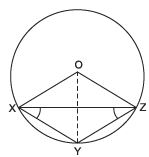∴ ∠XOY = 2∠XZY                …(1)
Similarly, ∠YOZ = 2∠YXZ                …(2)
Adding (1) and (2), we have ∠XOY + ∠YOZ = 2∠XZY + 2∠YXZ
⇒ ∠XOZ = 2[∠XZY + ∠YXZ]

Question 6. Show that the sum of the opposite angles of a cyclic quadrilateral is 180º.
Solution:
We have a cyclic quadrilateral. Let us join AC and BD. Since, angles in the same segment are equal.
and ∠BAC = ∠BDC                …(2)
Adding (1) and (2), we have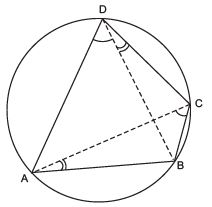∠ACB + ∠BAC = ∠ADB + ∠BDC
⇒ ∠ACB + ∠BAC = ∠ADC
Adding ∠ABC to both sides, we have ∠ACB + ∠BAC + ∠ABC = ∠ADC + ∠ABC
But, ∠ACB + ∠BAC + ∠ABC = 180º                 [Sum of the angles of ΔABC = 180º]
∴ ∠ADC + ∠ABC = 180º
⇒ ∠B + ∠D = 180º
Since, ∠A + ∠B + ∠C + ∠D = 360º
⇒ ∠A + ∠C = 360º ∠ 180º = 180º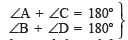Question 7. Prove that the quadrilateral formed by angle bisectors of a cyclic quadrilateral is also cyclic.
Solution:
We have a cyclic quadrilateral ABCD in which the bisectors ∠A, ∠B, ∠C and ∠D for a quadrilateral PQRS.
From ΔABP, we have ∠PAB + ∠PBA + ∠P = 180º                [Sum of the three angles of ΔABP]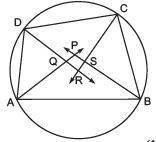⇒ (1/2) ∠A + (1/2)∠B + ∠P = 180º                …(1)
From ΔCDR, we have
∠RCD + ∠RDC + ∠R = 180º                [Sum of the three angles of ΔCDR.]
⇒ (1/2)∠C +(1/2)∠D + ∠R = 180°                 …(2)
Adding (1) and (2), we have (1/2)∠A + (1/2)∠B + (1/2)∠C +(1/2)∠D + ∠P + ∠R = 360º
⇒ (1/2) (∠A + ∠B + ∠C + ∠D) + ∠P + ∠R = 360º
⇒ (1/2)(360º) + ∠P + ∠R = 360º                 [∵ ∠A + ∠B + ∠C + ∠D = 360°]
⇒ ∠P + ∠R = 360º -(1/2) (360º) = 180º
Similarly, ∠Q + ∠S = 180º
Thus, the pairs of opposite angles of quadrilateral PQRS are supplementary.
Hence, PQRS is cyclic.

,

,

,

,

,

,

,

,

,

,

,

,

,

,

,

,

,

,

,

,

,

;